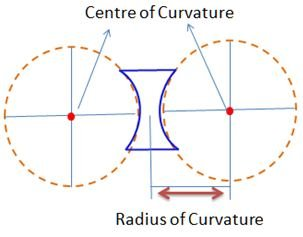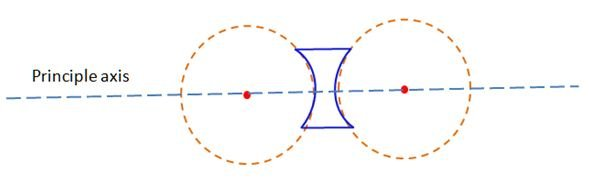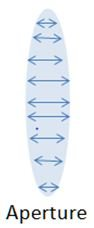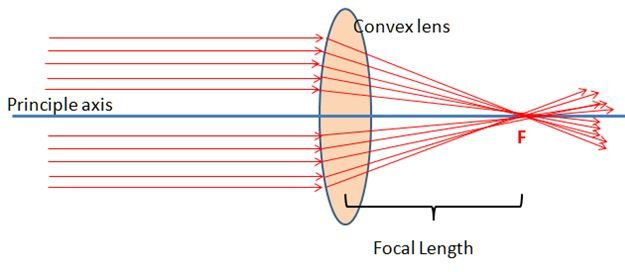# Definition Of Radius Of Curvature

## Definition Of Radius Of Curvature

The radius of the circle formed with the curved part of the lens is known as radius of curvature.

We draw a circle with the help of the curved part of the lens, and locate its centre, by measuring the radius of the circle from the centre we get radius of curvature. .#### Principle Axis:

As the lens contains two spherical surfaces it will have two centers of curvatures, A line joining these two centres and passing through the optical centre is called principal axis.#### Aperture:

Aperture is the effective of width of a lens through which refraction takes place.#### Principle Focus and Focal Length

When the rays of light passing parallel to the principle axis of a convex lens incident on a convex lens, the rays converge to meet at a point on the principle axis. This point is known as principle focus. For a concave lens when the diverged rays are produced backward they appear to meet at a point and this point is known as Principle focus.
In both the cases the distance between Principle focus and the optical centre is known as focal length.
Generally the focal length will be half of the radius of curvature.